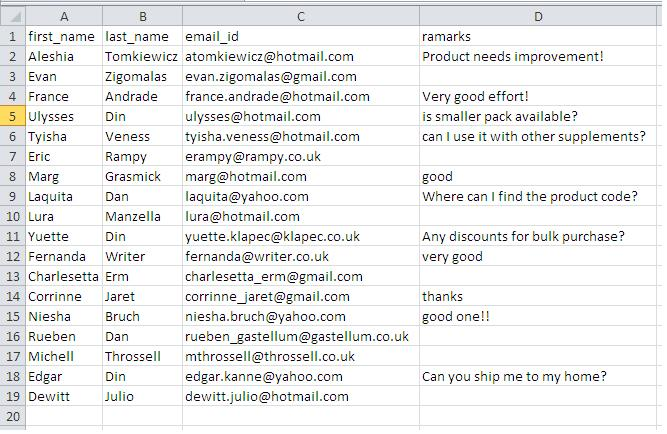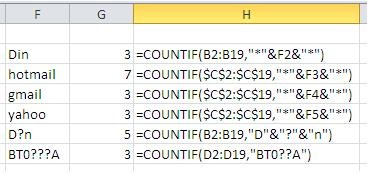# Using Wild Cards To Count Last Names – A Quick TutorialWhenever we have data, we want to extract meaningful information from it. One method of doing this is to filter the data for certain information and can revert to the use of advance filters to put multiple criteria to extract such information. Additionally we can also use wild cards to filter such information coupled with filtering and formulas.

Today we will learn a quick technique that is used to count data entries with text. We will learn how to use three wild card characters i.e. an asterisk (“*”) and a question mark (“?”).

Consider the following sample data and let’s take them one by one and see how they work:Asterisk Mark:

This symbol is used to count for the criteria for any number of characters at that position. Referring to the example sheet if we count the last_name with Din the answer comes out to be 3. Here we have used following formula to reach the result:

=COUNTIF(\$D\$2:\$D\$19,"*"&F1&"*")

Where F1 contains the text to be searched.

Another example could be to count the number of persons using certain email services for example number of person using yahoo is 3, number of users with Gmail id is also 3 where as 7 individuals are using Hotmail. The information is also extracted using the following formula:

=COUNTIF(\$D\$2:\$D\$19,"*"&F2&"*")Question Mark:

The question mark symbol is used to done a single positions and if used represents in that position. Using such a construction with COUNTIFS can tell us all the items with such pattern.

For example consider if you needed to count all the names that started with D and ended with n. The count would have been. The answer should have been 5 with using following formula:

=COUNTIF(B3:B19,"D"&"?"&"n")

Another example could be to count the emp_ids that has pattern of BT0???A. Task can be achieved by using multiple question marks like in the following formula:

=COUNTIF(D2:D19,"BT0??A")

Thus with the help of this simple trick we can extract valuable information with ease.